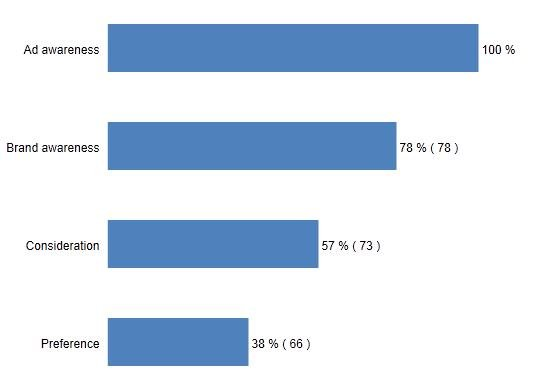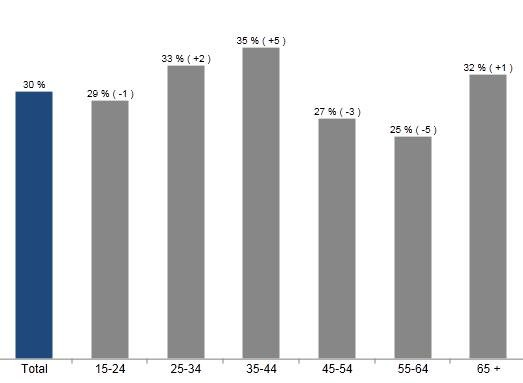The benchmark values can be based on items in the Legend or in the Axis. The user can select what calculation type to use when showing the difference between two values:

• Difference in percent units
• Percentage difference
• Conversion rates

The user can select if the comparison series should be hidden or not and also which values should be compared to each other:

• All previous data points (the only support in the previous version)
• The previous data point
• All next data points
• The next data point
• First data point
• Last data point

The image below shows a time column where each week is compared to the previous week. The difference is shown in percentage units.The image below shows a Brand Funnel with conversion rate.In the image below the chart compares each Age group against the Total. The difference is shown in percentage units.The setup is done in the Settings tab in the Chart/Table setup window. The setup is performed in five steps.

1. Define if the Benchmark calculation should be based on Legend or Axis items
2. Define which item in Legend or Axis that should be used
3. Define Calculation type
4. Define to which data point(s) the comparison should be performed
5. Define if the comparison data point should be hidden or not.

The calculation options possible here are:

• Difference in percentage units: VALUE Y – VALUE X
• Difference in percent shares: (VALUE Y – VALUE X)/VALUE X*100
• Conversion rate: VALUE Y/VALUE X*100